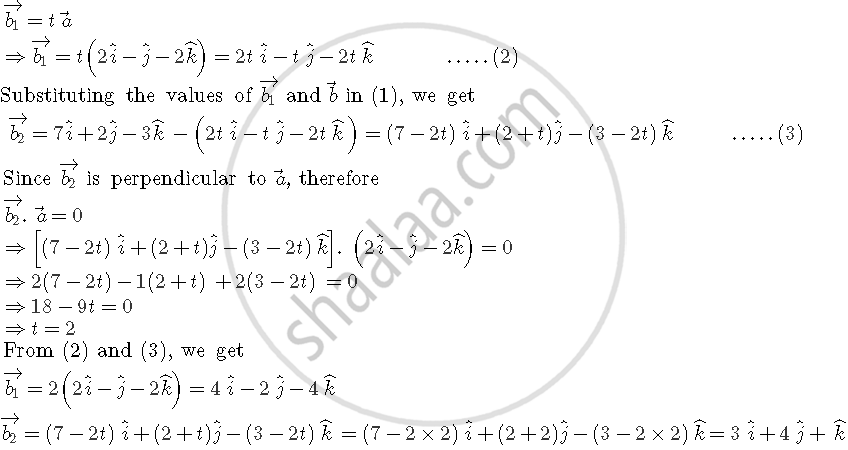Share

# If Veca = 2hati - Hatj - 2hatk "And" Vecb = 7hati + 2hatj - 3hatk, , Then Express Vecb in the Form of Vecb = Vecb_1 + Vecb_2, Where Vecb_1 is Parallel to Veca and Vecb_2 is Perpendicular to Veca - CBSE (Commerce) Class 12 - Mathematics

#### Question

if veca = 2hati - hatj - 2hatk " and " vecb = 7hati + 2hatj - 3hatk, , then express vecb in the form of vecb = vec(b_1) + vec(b_2), where vec(b_1)  is parallel to veca and vec(b_2) is perpendicular to veca

#### Solution

Let veca = 2hati - hatj - 2hatk " and " vecb = 7hati + 2hatj - 3hatk

Now, vec(b_1) and vec(b_2) are two vectors such that

vecb = vec(b_1) + vec(b_2)

=> vec(b_2) = vecb - vec(b_1)  ....(1)

Since vec(b_1)is parallel to veca, thereforeIs there an error in this question or solution?

#### Video TutorialsVIEW ALL 

Solution If Veca = 2hati - Hatj - 2hatk "And" Vecb = 7hati + 2hatj - 3hatk, , Then Express Vecb in the Form of Vecb = Vecb_1 + Vecb_2, Where Vecb_1 is Parallel to Veca and Vecb_2 is Perpendicular to Veca Concept: Types of Vectors.
S